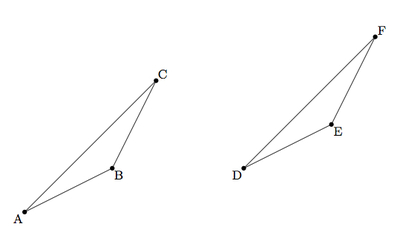# Identifying Translations

Alignments to Content Standards: G-CO.A.4

Suppose $\triangle DEF$ below can be obtained from $\triangle ABC$ by a translation:1. Explain why $ABED$, $ACFD$, and $BCFE$ are parallelograms.
2. Explain why $|AD| = |BE| = |CF|$.

## IM Commentary

The purpose of this task is to study the impact of translations on triangles. In the context of this problem, $\triangle DEF$ is the translate of $\triangle ABC$ by the segment $\overline{AD}$: students will visually understand this but the notion of forming parallelograms out of the vertices of $\triangle ABC$ and their translates will likely be new. The close link between translations of line segments and parallelograms is studied further in https://www.illustrativemathematics.org/illustrations/1511

One important observation concerning this task is that no parallelogram is formed in the case where the direction of translation is parallel to the segment being translated. In this case, the original points are collinear with their translates.

## Solution

1. We will show that $ACFD$ is a parallelogram. Analogous arguments (changing the vertices but not the steps of the argument) show that $ABED$ and $BCFE$ are parallelograms. Below is a picture of $ACFD$ with transverse $\overline{CD}$:We can show that $\triangle ACD$ is congruent to $\triangle FDC$ using SSS: $|AC| = |DF|$ because $\overline{DF}$ is the translated image of $\overline{AC}$ and translations preserve distance, $|AD| = |CF|$ since each vertex of $\triangle ABC$ is displaced by the same amount in the same direction to obtain $\triangle DEF$, and $|CD| = |DC|$. We conclude that $m(\angle CDA) = m(\angle DCF)$. This means that $\overleftrightarrow{AD}$ is parallel to $\overleftrightarrow{CF}$ since alternate interior angles of these lines, cut by transverse $\overleftrightarrow{CD}$, are congruent. We can also conclude that $m(\angle DCA) = m(\angle CDF)$ and this makes $\overleftrightarrow{AC}$ parallel to $\overleftrightarrow{DF}$ since alternate interior angles of these lines, again cut by transverse $\overleftrightarrow{CD}$, are congruent. We have shown that opposite sides of $ACFD$ are contained in parallel lines and so it is a parallelogram

2. We saw in the argument for part (a) why $\overline{AD}$, $\overline{BE}$ and $\overline{CF}$ are congruent. Each of these segments represents the displacement of a point in the plane to its image under translation. Translations, by their defintiion, displace every point in the plane by the same distance (in the same direction). This shows that $|AD|=|BE|=|DF|$.